# Mice

Mice consumed a circular hole in a slice of cheese. The cheese has the shape of a circular cut with a radius of 20 cm and an angle of 90 degrees. What percentage of the cheese ate mice if they made 20 holes with a diameter of 2 cm?

Correct result:

f =  20 %

#### Solution: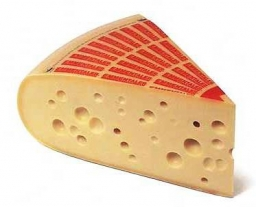We would be pleased if you find an error in the word problem, spelling mistakes, or inaccuracies and send it to us. Thank you!Tips to related online calculators
Need help calculate sum, simplify or multiply fractions? Try our fraction calculator.

#### You need to know the following knowledge to solve this word math problem:

We encourage you to watch this tutorial video on this math problem:

## Next similar math problems:

• Circle sectorCircular sector with a central angle 160° has area 452 cm2. Calculate its radius r.
• Circular sector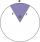I have a circular sector with a length 15 cm with an unknown central angle. It is inscribed by a circle with radius 5 cm. What is the central angle alpha in the circular sector?
• Annulus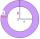Two concentric circles form an annulus of width 10 cm. The radius of the smaller circle is 20 cm. Calculate the content area of annulus.
• Circle arcCalculate the area of the circular arc in m2 where the diameter is 290 dm and a central angle is 135°. Result round to three decimal places.
• SectorThe perimeter of a circular sector with an angle 1.8 rad is 64 cm. Determine the radius of the circle from which the sector comes.
• CirclesThe areas of the two circles are in the ratio 2:20. The larger circle has a diameter 20. Calculate the radius of the smaller circle.
• Arc-sectorarc length = 17 cm area of sector = 55 cm2 arc angle = ? the radius of the sector = ?
• Equilateral triangle vs circle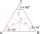Find the area of an equilateral triangle inscribed in a circle of radius r = 9 cm. What percentage of the circle area does it occupy?
• Sphere parts, segmentA sphere with a diameter of 20.6 cm, the cut is a circle with a diameter of 16.2 cm. .What are the volume of the segment and the surface of the segment?
• Cone A2VThe surface of the cone in the plane is a circular arc with central angle of 126° and area 415 cm2. Calculate the volume of a cone.
• Circular flowerbed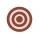Circular flowerbed with diameter 8 m we split by concentric circle to circle and annulus with the same area. Find the radius of the circle.
• Circle and angleWhat is the length of the arc of a circle with radius r = 207 mm with cental angle 5.33 rad?
• Circular arcCalculate the length of the circular arc if the diameter d = 20cm and the angle alpha = 142 °
• A spherical segment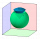A spherical section whose axial section has an angle of j = 120° in the center of the sphere is part of a sphere with a radius r = 10 cm. Calculate the cut surface.
• Two rectanglesI cut out two rectangles with 54 cm², 90 cm². Their sides are expressed in whole centimeters. If I put these rectangles together I get a rectangle with an area of 144 cm2. What dimensions can this large rectangle have? Write all options. Explain your calc
• Which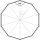Which of the following numbers most accurately area of a regular decagon with side s = 2 cm? (A) 9.51 cm2 (B) 20 cm2 (C) 30.78 cm2 (D) 31.84 cm2 (E) 32.90 cm2
• Cutting square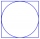From a square with a side of 30 cm, we cut the circle with the highest possible diameter. How many percents of the square content is this circle?